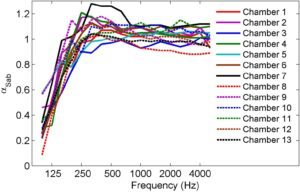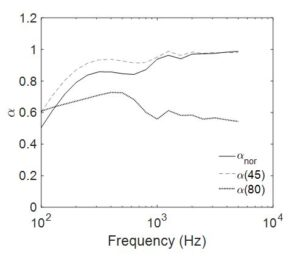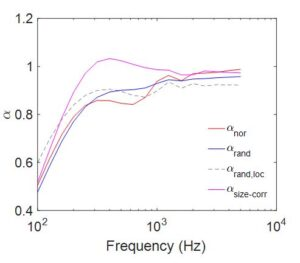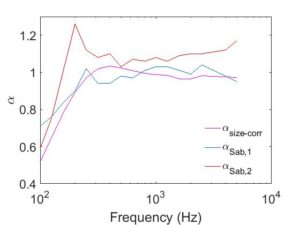Predicting sound absorption is in general troublesome. We have too many definitions; e.g. normal incidence, random incidence, field incidence, angle-dependent absorption coefficient, local-reaction or extended reaction absorption coefficient, etc.  Another problem is that there are various standardised methods, but none of them can give an absolute truth.

Cheol-Ho Jeong,  Associate Professor at DTU, was one of the keynote speakers at the latest BNAM conference that took place in Stockholm, where he discussed this particular topic, and we are very happy to be able to share with you this guest post by him with some of his thoughts and findings.

## Guest post by Cheol-Ho Jeong, Associate Professor at DTU

There are many versatile acoustic simulation tools for the UK’s (and world’s?) second weirdest job. Admittedly, we have no absolute best simulation method, as it really depends on a range of factors like:

• The purpose (design or renovation)
• Phenomena investigated (interference, diffraction, scattering, diffusion, absorption)
• Frequency range of interest (typically below or beyond the Schroeder frequency)
• More focus on the precision or calculation speed, etc.

There are two main schemes; wave-based simulations, known to be computationally demanding but more accurate, and geometrical acoustics simulations, known to be very fast but approximate at high frequencies, such as image source or ray-based simulation.

The boundary conditions are of utmost importance in all types of room acoustic simulations, but a big problem arises here. Acousticians or even absorber/scatter manufacturers don’t know exactly how much the surfaces absorb sound. There are various standardised methods, but none of them can give us the absolute truth.For example, a recent round-robin test of several absorbers according to the well-known reverberation chamber method, ISO 354, gives a surprisingly big inter-chamber variation because the reverberation chambers are non-diffuse in many different ways.

The impedance tube method, ISO 10354, gives more reliable results, but only when the samples are corrected fitted to the tubes and only for normal incidence conditions.

## A flora of definitions

There is yet another big problem with predictions of sound absorption. There are many empirical formulas and too many definitions, e.g., normal incidence, random incidence, field incidence, angle-dependent absorption coefficient, local-reaction or extended reaction absorption coefficient, etc. This blog article will now point out this problem.

## Example of a 10cm porous absorber

Let’s assume a 10 cm porous absorber backed by a 10 cm air cavity, which is quite common for ceiling absorbers. We, very luckily, know the density (27 kg/m3) or air flow resistivity, 12900 Nsm-4 (ISO 9053 specifies how to measure it) of the porous absorber, which is known to be well connected to the absorptive capability of fibrous absorbers. From this value, acoustic practitioners use their best knowledge on estimating the absorption coefficient or surface impedance values. Let’s look at some examples:### Varying absorption coefficients

First, depending on the angle of incidence, the absorption coefficients vary quite a lot. αnor indicates the normal incidence, α(45) from 45 degrees, α(80) from an almost grazing incidence angle.

Depending on other considerations, either local-reaction or extended reaction, random incidence or single incidence at the normal direction, there is also a relatively small variation as seen in the next figure. These three (αnor, αrand, αrand,loc) are calculated based on the assumption that the absorber of interest is infinitely large.

###Adjusting for size

However, in reality, no absorbers can be infinitely large, so an appropriate size correction is needed, e.g., Thomasson’s size correction assuming an infinitely large rigid baffle behind the absorber.

Then, the size-corrected absorption coefficient is quite different from the calculated absorption coefficient using the infinite panel assumption.

Random incidence is probably the most commonly used, it assumes a uniform distribution of sound incidence onto the absorber; which never ever happens in reality, unfortunately.

### Comparing calculated and measured absorption coefficientSo far, we discussed how the ‘calculated’ absorption coefficients differ depending on the assumptions. Now it is time to compare the measured and calculated absorption coefficient. Absorption measurements according to ISO 354 in two reverberation chambers are different, one, αSab,1, is somehow similar to the theoretical size-corrected absorption coefficient, but the other,αSab,2 is quite different.

So it must be confessed that answering, even vaguely, our students who want to know about how to determine or estimate the absorption characteristics of room surfaces for their weird acoustic projects, is very painful…

More scientific info can be found in a conference paper I’ve written together with Gerd Marbjerg, and Jonas Brunskog; “Uncertainty of input data for room acoustic simulations,” Baltic Nordic Acoustic Meeting (BNAM) 2016, June 2016, Stockholm, Sweden.